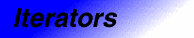# trivialCategory: iterators Component type: concept

## Description

A Trivial Iterator is an object that may be dereferenced to refer to some other object. Arithmetic operations (such as increment and comparison) are not guaranteed to be supported.

## Associated types

 Value type The type of the value obtained by dereferencing a Trivial Iterator

## Notation

 `X` A type that is a model of Trivial Iterator `T` The value type of `X` `x`, `y` Object of type `X` `t` Object of type `T`

## Definitions

A type that is a model of Trivial Iterator may be mutable, meaning that the values referred to by objects of that type may be modified, or constant, meaning that they may not. For example, `int*` is a mutable iterator type and `const int*` is a constant iterator type. If an iterator type is mutable, this implies that its value type is a model of Assignable; the converse, though, is not necessarily true.

A Trivial Iterator may have a singular value, meaning that the results of most operations, including comparison for equality, are undefined. The only operation that a is guaranteed to be supported is assigning a nonsingular iterator to a singular iterator.

A Trivial Iterator may have a dereferenceable value, meaning that dereferencing it yields a well-defined value. Dereferenceable iterators are always nonsingular, but the converse is not true. For example, a null pointer is nonsingular (there are well defined operations involving null pointers) even thought it is not dereferenceable.

Invalidating a dereferenceable iterator means performing an operation after which the iterator might be nondereferenceable or singular. For example, if `p` is a pointer, then `delete p` invalidates `p`.

## Valid expressions

In addition to the expressions defined in Assignable, EqualityComparable, and DefaultConstructible, the following expressions must be valid.

Name Expression Type requirements Return type
Default constructor `X x`
Dereference `*x`   Convertible to `T` 
Dereference assignment `*x = t` `X` is mutable
Member access `x->m`  `T` is a type for which `x.m` is defined

## Expression semantics

Name Expression Precondition Semantics Postcondition
Default constructor `X x`     `x` is singular
Dereference `*x` `x` is dereferenceable
Dereference assignment `*x = t` `x` is dereferenceable   `*x` is a copy of t
Member access `x->m` `x` is dereferenceable Equivalent to `(*x).m`

## Complexity guarantees

The complexity of operations on trivial iterators is guaranteed to be amortized constant time.

## Invariants

 Identity `x == y` if and only if `&*x == &*y`

## Models

• A pointer to an object that is not part of an array.